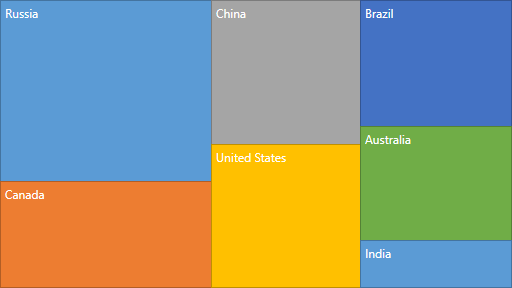20.2
20.1
19.2
19.1
18.2
18.1
17.2

# SquarifiedLayoutAlgorithm Class

A class representing the Squarified layout algorithm.

Namespace: DevExpress.Xpf.TreeMap

Assembly: DevExpress.Xpf.TreeMap.v19.1.dll

## Declaration

``````public class SquarifiedLayoutAlgorithm :
TreeMapLayoutAlgorithm``````
``````Public Class SquarifiedLayoutAlgorithm
Inherits TreeMapLayoutAlgorithm``````

## Remarks

The idea of the algorithm is to layout the rectangles along the required direction in the available display area as long as the highest width/height ratio of any rectangle in the current row keeps improving. Once the highest aspect ratio among the rectangles has reached a minimum, and adding another rectangle to the current row would increase the worst aspect ratio, the current row is fixed and a new row is created.

The following image demonstrates the tree map layout calculated using this algorithm.## Examples

To customize the layout algorithm, do the following.

##### NOTE

A complete sample project is available at https://github.com/DevExpress-Examples/how-to-change-the-layout-algorithm-used-by-treemap-t308988.

``````<dxtm:TreeMapControl x:Name="treeMap"
LayoutAlgorithm="{Binding ElementName=lbLayoutAlgorithm, Path=SelectedValue}">
<!-- endregion #LayoutAlgorithm-->
<dxtm:TreeMapControl.Colorizer>
<dxtm:TreeMapPaletteColorizer/>
</dxtm:TreeMapControl.Colorizer>
<dxtm:TreeMapItemStorage>
<dxtm:TreeMapItem Label="Americas">
<dxtm:TreeMapItem Label="United States" Value="17.418"/>
<dxtm:TreeMapItem Label="Brazil" Value="2.353"/>
</dxtm:TreeMapItem>
<dxtm:TreeMapItem Label="Europe">
<dxtm:TreeMapItem Label="Germany" Value="3.859"/>
<dxtm:TreeMapItem Label="United Kingdom" Value="2.945"/>
<dxtm:TreeMapItem Label="France" Value="2.846"/>
<dxtm:TreeMapItem Label="Italy" Value="2.147"/>
<dxtm:TreeMapItem Label="Russia" Value="1.857"/>
</dxtm:TreeMapItem>
<dxtm:TreeMapItem Label="Asia">
<dxtm:TreeMapItem Label="China" Value="10.380"/>
<dxtm:TreeMapItem Label="Japan" Value="4.616"/>
<dxtm:TreeMapItem Label="India" Value="2.049"/>
</dxtm:TreeMapItem>
</dxtm:TreeMapItemStorage>
<!--region #CloseTag-->
</dxtm:TreeMapControl>
<!--endregion #CloseTag-->
<Grid Grid.Column="1" Margin="4">
<Grid.RowDefinitions>
<RowDefinition Height="Auto"/>
<RowDefinition Height="Auto"/>
<RowDefinition Height="Auto"/>
<RowDefinition Height="Auto"/>
</Grid.RowDefinitions>
<TextBlock Grid.Row="0"
Margin="0,0,0,4"
Text="Layout Algorithm:"/>
<!--region #LayoutAlgorithmList-->
<ListBox x:Name="lbLayoutAlgorithm"
Grid.Row="1"
DisplayMemberPath="LayoutAlgorithmName"
SelectedValuePath="LayoutAlgorithm"
SelectedIndex="0">
<local:LayoutAlgorithmWarapper LayoutAlgorithmName="Slice and Dice">
<dxtm:SliceAndDiceLayoutAlgorithm/>
</local:LayoutAlgorithmWarapper>
<local:LayoutAlgorithmWarapper LayoutAlgorithmName="Squarified">
<dxtm:SquarifiedLayoutAlgorithm/>
</local:LayoutAlgorithmWarapper>
<local:LayoutAlgorithmWarapper LayoutAlgorithmName="Striped">
<dxtm:StripedLayoutAlgorithm/>
</local:LayoutAlgorithmWarapper>
</ListBox>
``````